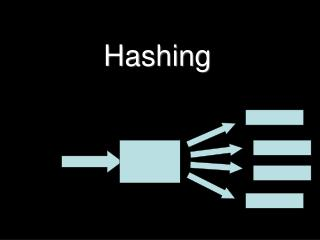Download PresentationHashing

# Hashing - PowerPoint PPT PresentationDownload Presentation## Hashing

- - - - - - - - - - - - - - - - - - - - - - - - - - - E N D - - - - - - - - - - - - - - - - - - - - - - - - - - -
##### Presentation Transcript

1. Hashing

2. What is hashing • Simply, generating a numeric key using an algorithm (hash function) • Definition: A function that maps keys to integers, usually to get an even distribution on a smaller set of values. • The very simplest hash function is to use the modulus operator % • Input range % key range

3. Input and key range • Example. We want to store 7 digit telephone numbers so that they can be quickly retrieved. • Number of expected entries = 100 • Range of telephone numbers = 0 – 9999999 Simple hashing algorithm hash = inputNumber % 100 What’s the effect?

4. Applications of hashing • File management – working out where to store records • Comparing complex values • Cryptography – creating digital signatures – eg: md5

5. Collisions • Where the hash value returned for two keys is the same. • What to do? • Open hashing • Closed hashing • Deleting • The 2/3rds rule

6. DJB Hash function • “An algorithm produced by Professor Daniel J. Bernstein and shown first to the world on the usenet newsgroup comp.lang.c. It is one of the most efficient hash functions ever published. “ def DJBHash(key): hash = 5381 for i in range(len(key)): hash = ((hash << 5) + hash) + ord(key[i]) returnhash